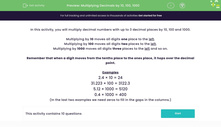# Multiplying Decimals by 10, 100, 1000

In this worksheet, students multiply decimal numbers up to 3 decimal places by 10, 100 and 1000.Key stage:  KS 2

Curriculum topic:   Number: Fractions, Decimals and Percentages

Curriculum subtopic:   Use Three Decimal Places

Difficulty level:#### Worksheet Overview

In this activity, you will multiply decimal numbers with up to 3 decimal places by 10, 100 and 1000.

Multiplying by 10 moves all digits one place to the left.

Multiplying by 100 moves all digits two places to the left.

Multiplying by 1000 moves all digits three places to the left and so on.

Remember that when a digit moves from the tenths place to the ones place, it hops over the decimal point.

Examples

2.4 × 10 = 24

31.223 × 100 = 3122.3

5.12 × 1000 = 5120

0.4 × 1000 = 400

(In the last two examples we need zeros to fill in the gaps in the columns.)

### What is EdPlace?

We're your National Curriculum aligned online education content provider helping each child succeed in English, maths and science from year 1 to GCSE. With an EdPlace account you’ll be able to track and measure progress, helping each child achieve their best. We build confidence and attainment by personalising each child’s learning at a level that suits them.

Get started